# PSAT Math : Percentage

## Example Questions

1 2 3 4 5 6 7 8 10 Next →

### Example Question #2 : How To Find The Part From The Whole With Percentage

Three salesmen, Gor, Levon, and Raffi, competed to sell the highest number of cars in the month of August. A total of 250 cars were sold.

Gor sold 100 cars. Levon sold 62% of the remaining cars, and Raffi sold the rest.

How many cars did Raffi sell?

39

57

75

77

93

57

Explanation:

We first subtract the 100 cars that Gor sold from the total of 250 sold. We are left with 150 cars, and we know that Levon sold 62% of them. 100% – 62% = 38%. Hence, Raffi sold 38% of 150 cars. 150 * 0.38 = 57

### Example Question #91 : Percentage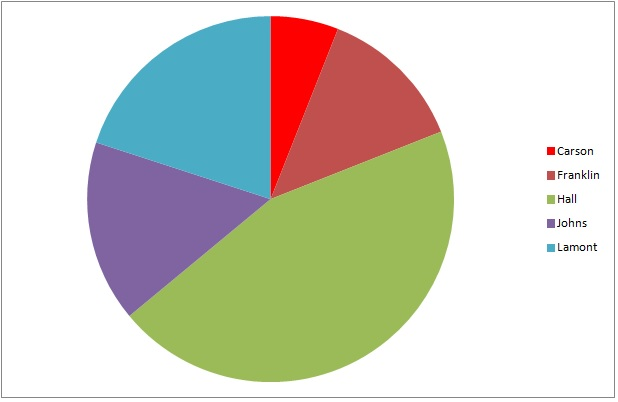The above graph represents the results of a general election for mayor of Washington City. Hall is a Republican; Johns is a Democrat;  the other three are independents.

5,328 people voted for independent candidates. Which is the best estimate for the number of people who voted for Hall?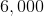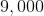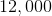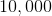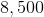Explanation:

The green wedge, which represents Hall, is about 45% of the circle. The three wedges representing independents Carson, Franklin, and Lamont - dark red, bright red, and turquoise, respectively - together comprise about 40% of the circle. We can set up, and solve for the unknown in, a proportion statement as follows: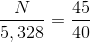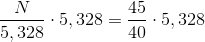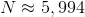The best estimate of the five is 6,000.

### Example Question #1 : How To Find The Part From The Whole With PercentageThe above graph represents the results of a general election for two seats on the city council of Lincoln City. Frankllin and Hall are Democrats; Johns and Lamont are Republicans; Carson is an independent.

6,219 people voted for Republicans. Which of the following is the best estimate for the number of people who voted for Democrats?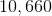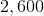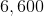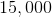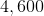Explanation:

The two wedges that represent the Democratic candidates - green (Hall) and dark red (Franklin) - together make up about 60% of the circle. The two wedges that represent the Republican candidates - purple (Johns) and blue (Lamont) - make up about 35% of the circle. We can estimate the number of people who voted for a Democrat using a proportion statement: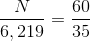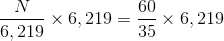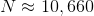10,660 is the best estimate.

### Example Question #1 : Whole And Part

A school is made up of 400 students. Each student must belong to one of the four clubs shown in the chart below. How many students are in chorus?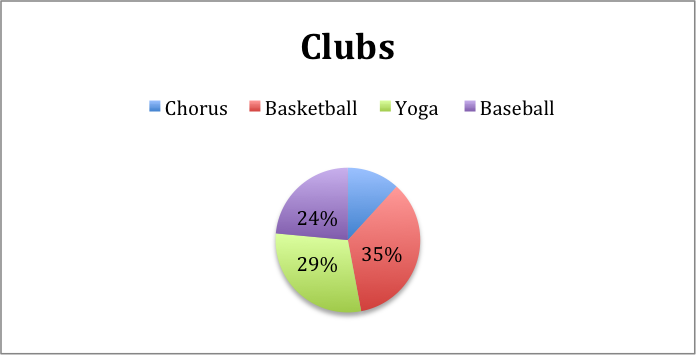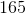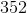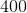Explanation:

You must first determine the percentage of the students in chorus. To do this, subtract the other percentages from 100: 100 – (35 + 29 + 24) = 12.

Therefore, 12% of the 400 students at this school attend chorus.

To determine the number of students in the chorus, multiply .12 (12%) by 400.

0.12(400) = 48

1 2 3 4 5 6 7 8 10 Next →

### All PSAT Math Resources